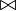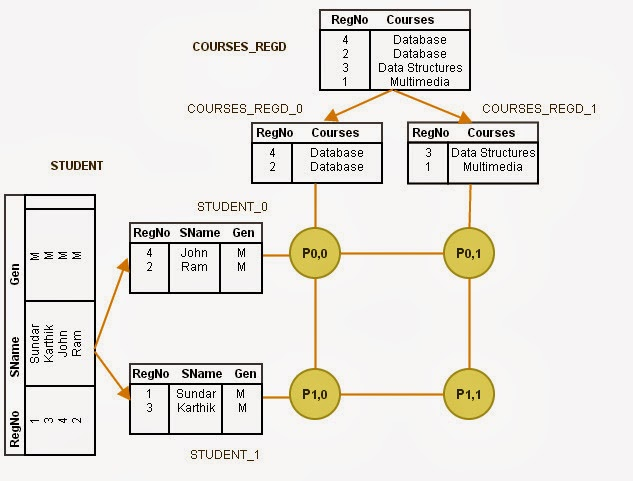## TOPICS (Click to Navigate)

Please visit, subscribe and share 10 Minutes Lectures in Computer Science

## Fragment and Replicate Join

#### Introduction

All of us know about different types of joins in terms of the nature of the join conditions and the operators used for join. They are Equi-join and Non-Equi Join. Equi-join is performed through checking an equality condition between different joining attributes of different tables. Non-equi join is performed through checking an inequality condition between joining attributes.
Equi-join is of the form,
SELECT columns FROM list_of_tables WHERE table1.column = table2.column;
whereas, Non-equi join is of the form,
SELECT columns FROM list_of_tables WHERE table1.column < table2.column;
(Or, any other operators >, <>, <=, >= etc. in the place of < in the above example)

We have discussed Partitioned Join in the previous post, where we partitioned the relational tables that are to be joined, into equal partitions and we performed join on individual partitions locally at every processor. Partitioning the relations on the joining attribute and join them will work only for joins that involve equality conditions.
Clearly, joining the tables by partitioning will work only for Equi-joins or natural joins. For inequality joins, partitioning will not work. Consider a join condition as given below;
r$\bowtie$r.a>s.b s
In this non-equal join condition, the tuples (records) of r must be joined with records of s for all the records where the value of attribute r.a is greater than s.b. In other words, all records of r join with some records of s and vice versa. That is, one of the relations’ all records must be joined with some of the records of other relation. For clear example, see Non-equi join post.

#### What does fragment and replicate mean?

Fragment means partitioning a table either horizontally or vertically (Horizontal and Vertical fragmentation). Replicate means duplicating the relation, i.e, generating similar copies of a table. This join is performed by fragmenting and replicating the tables to be joined.

### (How does Asymmetric Fragment and Replicate Join work?)

It is a variant of Fragment and Replicate join. It works as follows;
1. The system fragments table r into n fragments such that r0, r1, r2, .., rn-1, where r is one of the tables that is to be joined and n represents the number of processors. Any partitioning technique, round-robin, hash or range partitioning could be used to partition the relation.
2. The system replicates the other table, say s into n processors. That is, the system generates n copies of s and sends to n processors.
3. Now we have, r0 and s in processor P0,  r1 and s in processor P1, r2 and s in processor P2, .., rn-1 and s in processor Pn-1. The processor Pi is performing the join locally on ri and s.
Figure 1 given below shows the process of Asymmetric Fragment-and-Replicate join (it may not be the appropriate example, but it clearly shows the process);Figure 1 - process of Asymetric Fragment and Replicate Parallel Join

#### Points to Note:

1. Non-equal join can be performed in parallel.
2. If one of the relations to be joined is already partitioned into n processors, this technique is best suited, because we need to replicate the other relation.
3. Unlike in Partitioned Join, any partitioning techniques can be used.
4. If one of the relations to be joined is very small, the technique performs better.

### (How does Fragment and Replicate Join work?)

It is the general case of Asymmetric Fragment-and-Replicate join technique. Asymmetric technique is best suited if one of the relations to be joined is small, and if it can fit into memory. If the relations that are to be joined are large, and the joins is non-equal then we need to use Fragment-and-Replicate Join. It works as follows;
1. The system fragments table r into m fragments such that r0, r1, r2, .., rm-1, and s into n fragments such that s0, s1, s2, .., sn-1 . Any partitioning technique, round-robin, hash or range partitioning could be used to partition the relations.
2. The values for m and n are chosen based on the availability of processor. That is, we need at least m*n processors to perform join.
3. Now we have to distribute all the partitions of r and s into available processors. And, remember that we need to compare every tuple of one relation with every tuple of other relation. That is the records of r0 partition should be compared with all partitions of s, and the records of partition s0 should be compared with all partitions of r. This must be done with all the partitions of r and s as mentioned above. Hence, the data distribution is done as follows;
a. As we need m*n processors, let us assume that we have processors P0,0, P0,1, …, P0,n-1, P1,0, P1,1, …, Pm-1,n-1. Thus, processor Pi,j performs the join of ri with sj.
b. To ensure the comparison of every partition of r with every other partition of s, we replicate ri with the processors, Pi,0, Pi,1, Pi,2, …, Pi,n-1, where 0, 1, 2, …, n-1 are partitions of s. This replication ensures the comparison of every ri with complete s.
c. To ensure the comparison of every partition of s with every other partition of r, we replicate si with the processors, P0,i, P1,i, P2,i, …, Pm-1,i, where 0, 1, 2, …, m-1 are partitions of r. This replication ensures the comparison of every si with complete r.
4. Pi,j computes the join locally to produce the join result.
Figure 2 given below shows the process of general case Fragment-and-Replicate join (it may not be the appropriate example, but it clearly shows the process);Figure 2 - process of general case Fragment-and-Replicate Join

#### Points to Note:

1. Asymmetric Fragment-and-replicate join is the special case of general case Fragment-and-replicate join, where n or m is 1, i.e, if one of the relation does not have partitions.

2. When compared to asymmetric technique, Fragment-and-replicate join reduces the size of the tables at every processor.

3. Any partitioning techniques can be used and any joining technique can be used as well.

4. Fragment-and-replicate technique suits both Equi-join and Non-equi join.

5. It involves higher cost in partitioning.

An appropriate example will be discussed later.

### Related Posts:

Intraoperation Parallelism

Partitioned Parallel Join
Partitioned Parallel Hash-Join
Parallel Nested Loop Join

 Try to learn something about everything and everything about something. Thomas Huxley

## Featured Content

### Multiple choice questions in Natural Language Processing Home

MCQ in Natural Language Processing, Quiz questions with answers in NLP, Top interview questions in NLP with answers Multiple Choice Que...